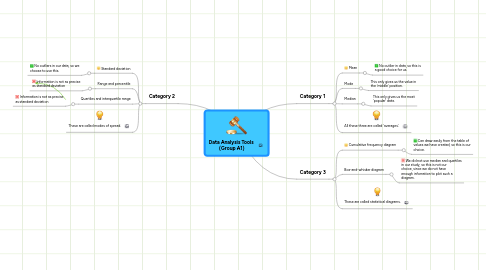# Data Analysis Tools (Group A1)

Get Started. It's FreeData Analysis Tools (Group A1)## 1. Category 1

### 1.1. Mean

1.1.1. No outlier in data, so this is a good choice for us.

### 1.2. Mode

1.2.1. This only gives us the value in the 'middle' position.

### 1.3. Median

1.3.1. This only gives us the most 'popular' data.

## 2. Category 2

### 2.1. Standard deviation

2.1.1. No outliers in our data, so we choose to use this.

### 2.2. Range and percentile

2.2.1. Information is not as precise as standard deviation

### 2.3. Quartiles and interquartile range

2.3.1. Information is not as precise as standard deviation

## 3. Category 3

### 3.1. Cumulative frequency diagram

3.1.1. Can draw easily from the table of values we have created, so this is our choice.

### 3.2. Box-and-whisker diagram

3.2.1. We did not use median and quartiles in our study, so this is not our choice, since we do not have enough infomation to plot such a diagram.